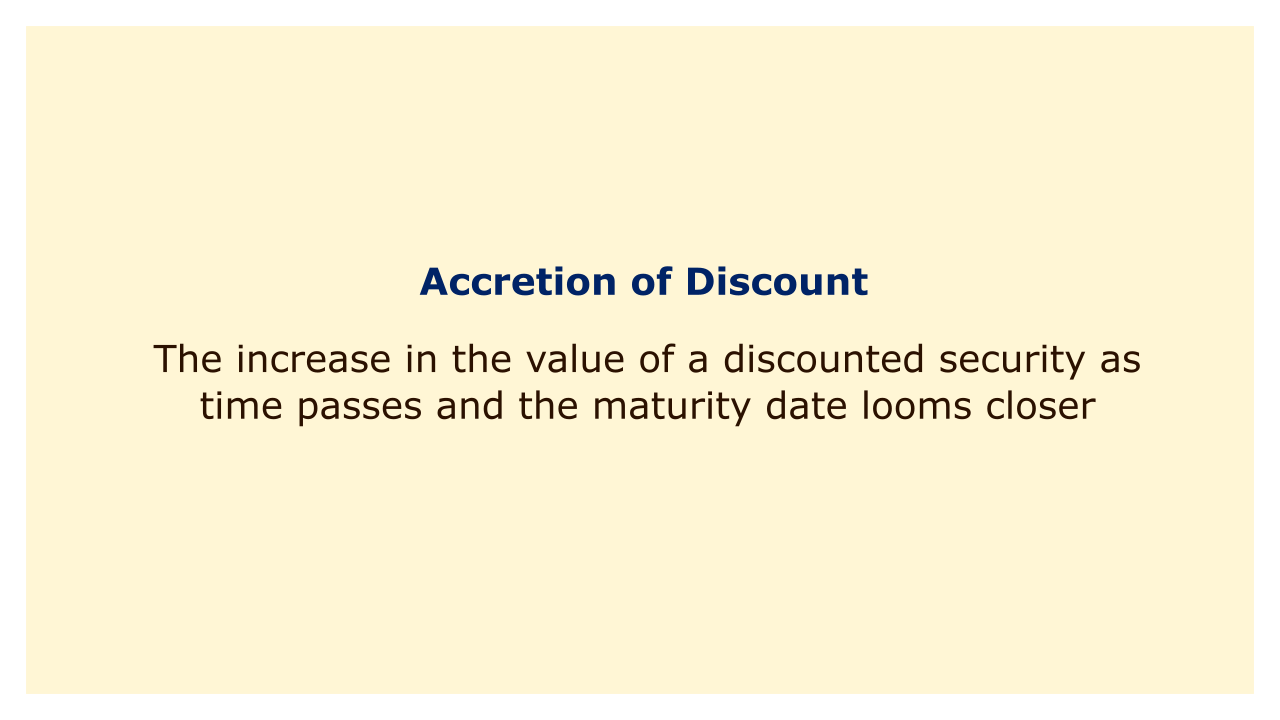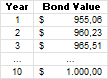# Accretion of DiscountImage: Moneybestpal.com

### Accretion of discount is the increase in the value of a discounted security as time passes and the maturity date looms closer. Bonds that are sold or bought below their face value are referred to as discounted securities. The amount that the issuer will give the bondholder upon maturity is known as the face value.

As an illustration, let's say you pay \$900 for a bond with a \$1,000 face value. As a result, you made a payment that was \$100 less than what you will be reimbursed when the loan matures. The discount is the name for this discrepancy. The bond's value will gradually rise as the maturity date approaches until it reaches its face value of \$1,000. Accrual of discount is the term used to describe this increase in value.

The accretion of discount is a reflection of the fact that the yield of a bond is influenced by both the coupon rate and the price at which it was purchased. The annual interest rate that the bondholder receives from the issuer is known as the coupon rate. The cost an investor pays to purchase a bond on the secondary market is known as the purchasing price.

Conversely, a larger return results from a reduced buying price. This is due to the fact that the investor will make a greater return on their investment thanks to interest income. For instance, if a bond has a \$1,000 face value, a 5% coupon rate, and an annual interest payment of \$50, it will earn \$50. You will receive a 5% yield if you purchase this bond at par, or at its face value. On the other hand, if you purchase this bond at a discount, or below its face value, your yield will be more than 5%. Due to the fact that you purchased the bond for less than \$1,000, you will consistently earn \$50 in interest.

The discrepancy between the yield and the coupon rate is represented by the accretion of discount. It is a method of accounting used to change the value of a bond that was purchased at a discounted price. It also has an impact on the annual reporting requirements for the bondholder's taxable income.

### How Is Accretion of Discount Calculated?

The straight-line method and the constant yield method are the two basic approaches for figuring out the accretion of discount.

#### The Straight-Line Method

The simplest technique to determine the accretion of discount is to use the straight-line method. It is assumed that the value rise will be dispersed equally across the bond's whole term. To use this method, you need to know:
• The purchase price of the bond
• The face value of the bond
• The term of the bond

The formula for accretion of discount using the straight-line method is:

Accretion Amount = (Face Value - Purchase Price) / Term

For example, suppose you buy a 10-year bond with a face value of \$1,000 at a price of \$900. Using the straight-line method, you can calculate the accretion amount as follows:

Accretion Amount = (\$1,000 - \$900) / 10

Accretion Amount = \$10

This means that every year, you will add \$10 to the value of your bond until it reaches its face value at maturity. Your bond's value will increase as follows:

Using this method, you will also report \$10 as taxable income every year.

#### The Constant Yield Method

The accretion of discounts can be calculated more precisely using the constant yield approach. The value growth is predicated on the bond's yield to maturity (YTM), which is a supposition. The yield to maturity (YTM) is the return that will be received on a bond kept to its maturity.

To use this method, you need to know:
• The purchase price of the bond
• The face value of the bond
• The coupon rate of the bond
• The term of the bond
• The YTM of the bond

The formula for accretion of discount using the constant yield method is:

Accretion Amount = Purchase Basis x (YTM / Accrual Periods per Year) - Coupon Interest

The purchase basis is the initial value of the bond plus the accumulated accretion. The number of times interest is paid annually is determined by the annual accrual periods. The amount of interest that the bond pays each accrual period is known as the coupon interest.

Consider purchasing a 10-year bond for \$950 that has a face value of \$1,000, a coupon rate of 5%, and a yield to maturity (YTM) of 6%. Semi-annual interest is paid on the bond. Using the constant yield method, you can calculate the accretion amount for the first year as follows:

Accretion Amount = \$950 x (0.06 / 2) - \$25

Accretion Amount = \$2.50

This means that for the first six months, you will add \$2.50 to the value of your bond and report it as taxable income. Your bond's value will increase to \$952.50.

For the next six months, you will use the new purchase basis of \$952.50 to calculate the accretion amount as follows:

Accretion Amount = \$952.50 x (0.06 / 2) - \$25

Accretion Amount = \$2.56

This means that for the second six months, you will add \$2.56 to the value of your bond and report it as taxable income. Your bond's value will increase to \$955.06.

You will repeat this process until the bond reaches its face value at maturity. Your bond's value will increase as follows:Image: Moneybestpal.com

Using this method, you will report a varying amount of taxable income every year, depending on the accretion amount.

### Why Is Accretion of Discount Important?

Accretion of discount is important for bond investors for several reasons:
• It has an impact on a bond's return on investment (ROI). An investor can obtain a yield that is more than the coupon rate by purchasing a bond at a discount. The additional income that the investor will get at maturity is represented by the discount that has accrued.
• It has an impact on a bondholder's tax obligations. The Internal Revenue Service (IRS) considers the accrual of discounts to be interest income, which must be declared on Form 1099-INT. Even though they do not get any cash payments from the bond until it matures, the bondholder is still required to pay taxes on the accretion amount each year.
• It has an impact on a bond's market value. A bond's market price will rise in tandem with the bond's value as a result of the accumulation of discount. As a result, depending on the market price at the time of sale, an investor who sells a bond before it matures may experience a financial gain or loss.
Tags